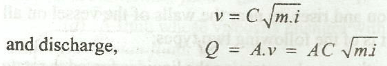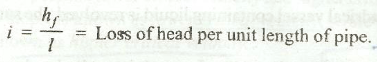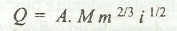# Flow Through Open Channels

Flow Through Open Channels - Describe the flow of a fluid through open channel. What is the formula to calculate flow and discharge through open channel.

According to Chezy formula, the mean velocity of liquid,where

C = Chezy's constant,

A = Area of flow,

m = Hydraulic mean depth
= Area of flow (A) / Wetted perimeter (P)
= d/4, for circular pipeAccording to Manning's formula, discharge through an open channel,where
M = Manning's constant.